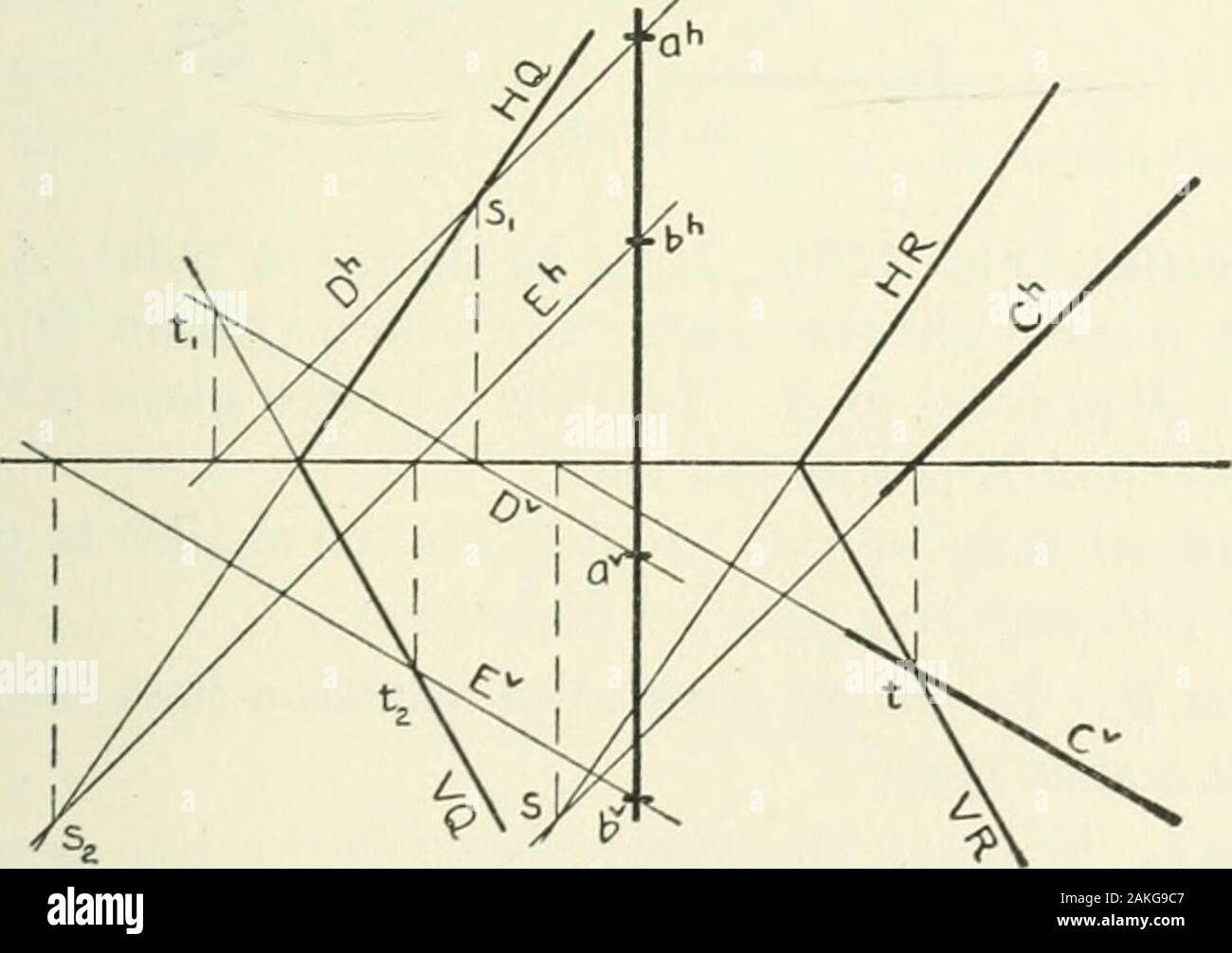# Descriptive geometry . Fig. 144. X, § 107] PARALLEL LINES AND PLANES 85 Special Case I. Suppose the second line is parallel to IIor V; then no auxiliary line is needed. Thus, in Fig. 144, letus find the plane which contains the line A and is parallel toB. The .ff-trace of any plane which is parallel to B must beparallel to Bh (§ 104). Hence, find the traces of A. DrawHQ through sx parallel to Bh; draw VQ through tx and thepoint in which HQ intersects GL. Special Case II. Suppose that either the first or the secondgiven line is a profile line. The general solution will apply tothis case ; but i## Image details

Contributor:

The Reading Room / Alamy Stock Photo

Image ID:

2AKG9C7

File size:

Releases:

Model - no | Property - noDo I need a release?

Dimensions:

1883 x 1328 px | 31.9 x 22.5 cm | 12.6 x 8.9 inches | 150dpi

This image is a public domain image, which means either that copyright has expired in the image or the copyright holder has waived their copyright. Alamy charges you a fee for access to the high resolution copy of the image.

This image could have imperfections as it’s either historical or reportage.

Descriptive geometry . Fig. 144. X, § 107] PARALLEL LINES AND PLANES 85 Special Case I. Suppose the second line is parallel to IIor V; then no auxiliary line is needed. Thus, in Fig. 144, letus find the plane which contains the line A and is parallel toB. The .ff-trace of any plane which is parallel to B must beparallel to Bh (§ 104). Hence, find the traces of A. DrawHQ through sx parallel to Bh; draw VQ through tx and thepoint in which HQ intersects GL. Special Case II. Suppose that either the first or the secondgiven line is a profile line. The general solution will apply tothis case ; but if the problem be solved in this manner, a profileprojection will be necessary. A simple construction, by whichthe use of a profile projection may be avoided, is as follows : Let ab and C, Fig. 145, be the given lines. Through onepoint of the profile line, as a, draw an auxiliary line D parallelto the line C; through the other point, b, of ab, draw a second. Fig. 145. auxiliary line E parallel to C. Pass the plane Q through thetwo parallel lines D and E. Then if the required plane is tocontain ab and be parallel to C, plane Q is the required plane.If, however, the required plane is to contain C and be parallelto ab, the plane It, passed through C parallel to plane Q (§ 103), is the required plane. 86 DESCRIPTIVE GEOMETRY [X, § 107 Problem 8. To find the plane which contains a given point and isparallel to each of two given lines. Analysis. Through the given point draw two auxiliary lines, one parallel to one given line, the other parallel to the othergiven line. The required plane is determined by the two aux-iliary lines.

Save up to 70% with our image packs